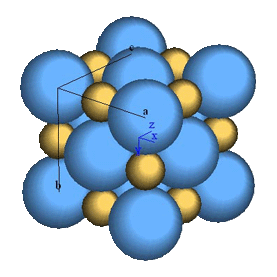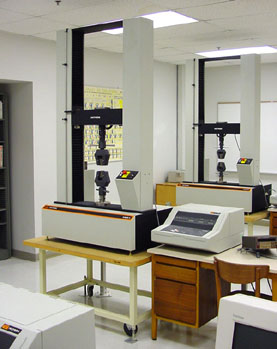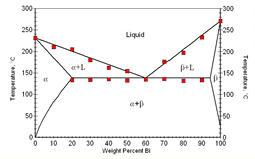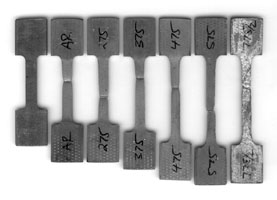# Introductory Materials Science Experiments

This page offers four experiments that cover classic topics taught in many introductory materials science laboratory courses. The only prerequisites for doing them are general chemistry and physics courses.The NaCl-type (Halite) structure.

1. Ionic Bonding
This experiment is a foray into computational chemistry in which students create a spreadsheet that computes the lattice energies and forces, equilibrium ionic spacing, elastic moduli, and melting point for at least two NaCl-type materials. The main point of the experiment is to become more familiar with the nature of the chemical bond and its relationship to a selected macroscopic properties.

• Presentation - an introduction to the experiment.  The same presentation that was given in the discussion session. (6.35 MB)
• Notes - a notes printout of the Power Point presentation used to introduce this topic in the discussion session. (Version with a white background.)
• Preparation - an introductory exercise that involves performing a few preliminary calculations before coming to the laboratory to attempt the full modeling exercise.
• Procedure - the complete procedure for the ionic bonding experiment.
• Report - recommendations for organizing and presenting the data from the experiment and writing the laboratory report.The Instron 4204 universal testing systems used in this experiment.

2. Mechanical Properties
This experiment covers the basics of stress and strain and the common mechanical properties one can measure using routine hardness and tensile tests. Several common alloys are tested and several different types of stress-strain behavior are observed. Materials tested include low-carbon steel (cold worked and annealed), OFHC copper, and 2024, 6061,7075 aluminum alloys.

• Presentation - A Power Point presentation that introduces the experiment. This is the same presentation that was given in the discussion session. (10.0 MB)
• Notes - a notes printout of the above Power Point presentation. (Version with a white background.)
• Preparation - an introductory exercise that involves looking up the mechanical properties of the materials that will be examined during the experiment. The data is plotted in a bar chart, making it easy to compare the properties of the different materials.
• Procedure - the complete procedure for the mechanical properties experiment.
• Report - recommendations for organizing and presenting the data from the experiment, such as stress-strain curves and bar charts, and writing the laboratory report.Typical results from the Bi/Sn equilibrium phase diagram experiment.

3. The Bi-Sn Equilibrium Phase Diagram
In this experiment the students measure the melting points and transformation temperatures for various compositions of bismuth and tin and use this information to construct an equilibrium phase diagram.

• Presentation - A Power Point presentation that introduces the experiment. This is the same presentation that was given in the discussion session. (17.0 MB)
• Notes - a notes printout of the above Power Point  presentation.
• Preparation - introductory exercise that involves preparing data tables and figures that are needed during and after the experiment.
• Data - data file that can be used to create a Bi/Sn equilibrium phase diagram using a spreadsheet.
• Procedure - the complete procedure for the Bi-Sn phase diagram experiment.
• Report - recommendations for organizing and presenting the data from the experiment and writing the laboratory report. The tables and graph generated in the preparatory exercise can be adapted for use in the final report.Tensile tested samples of 70/30 brass.

4. Recovery, Recrystallization, and Grain Growth
The students tensile test heat treated samples of brass to determine how heat treating influences the mechanical properties. Then they examine the microstructures and draw conclusions regarding the relationship between structure, processing and properties.

• Presentation - A Power Point presentation that introduces the experiment.  This is the same presentation that was given in the discussion session. (6.38 MB)
• Notes - a notes printout of the above Power Point presentation.
• Preparation - an introductory exercise that involves looking up the mechanical properties of the alloy that will be examined during the experiment. The data is plotted using an x-y graph that has a second y axis. This graph illustrates how different properties are altered by cold working and annealing.
• Procedure - the complete procedure for the recovery, recrystallization and grain growth experiment.
• Microstructures - the optical micrographs showing the longitudinal and transverse views of the microstructures of the samples tested during this experiment.